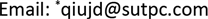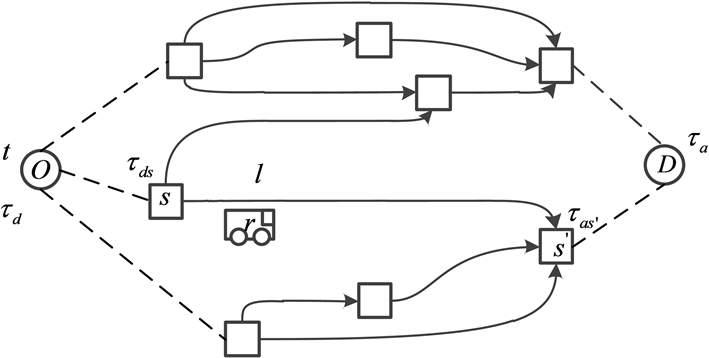﻿ 基于随机理论的公交路径选择建模 Modeling of Path Choice via Public Transit Based on the Random Theory

Modeling and Simulation
Vol.07 No.03(2018), Article ID:26649,9 pages
10.12677/MOS.2018.73021

Modeling of Path Choice via Public Transit Based on the Random Theory

Jiaxian Liang1*, Jiandong Qiu1, Jiajie Pan2

1Shenzhen Urban Transport Planning Center Co., Ltd., Shenzhen

2Traffic Information Engineering & Technology Research Center of Guangdong Province, ShenzhenReceived: Aug. 10th, 2018; accepted: Aug. 22nd, 2018; published: Aug. 29th, 2018ABSTRACT

The paper presents the analysis of the stop choice and bus choice behaviors in space-time dimension using the public transit path choice model under the framework of random utility models, based on the idea of hierarchical information processing. The parameters of the perceived utility function of bus choice are calibrated and tested from TIYUZHONGXIN BRT stop to DONGPUZHEN BRT stop (8 direct routes) in Guangzhou with IC data and bus arriving data in case study. The result shows that waiting time, on-board time, comfortable at stop, comfortable at bus and the on-board experience are statistically significant in bus choice model under the hierarchical processing of degree of comfort.

Keywords:Transit Path Choice Models, Perceived Utility, Behavioral Information Processing, Parameter Calibration

1深圳市城市交通规划设计研究中心有限公司，深圳

2广东省交通信息工程技术研究中心，深圳Copyright © 2018 by authors and Hans Publishers Inc.1. 引言

2. 公交路径选择模型Figure 1. Path example

2.1. 路径选择集

2.2. 路径选择机理

${V}_{s}\left[{\tau }_{d},t\right]={\beta }_{s}\cdot {X}_{s}+{{\beta }^{\prime }}_{s}\cdot {H}_{s}+{\beta }_{i_s}\cdot {X}_{i_s}+{\epsilon }_{s}$ (1)

${V}_{r}\ge {V}_{{r}^{\prime }},\forall {\tau }_{{r}^{\prime }}>{\tau }_{r}且r,{r}^{\prime }\in {R}_{s}\left[{\tau }_{r}\right]$ (2)

${V}_{r}={\beta }_{tb}\cdot T{B}_{r}+{\beta }_{tw}\cdot T{W}_{r}+{\beta }_{cf_s}\cdot C{F}_{s}+{\beta }_{cf_r}\cdot C{F}_{r}+{\beta }_{tf}\cdot T{F}_{r}+{\beta }_{i_l}\cdot {X}_{i_l}+{\epsilon }_{r}$ (3)

2.3. 学习过程

1) 恒定属性 ${X}^{c}$ ，属性值不随时间而改变，如换乘次数 $T{F}_{r}^{c}$ ，站点固定属性 ${X}_{s}^{c}$

2) 瞬时属性 ${X}^{te}$ ，这类属性是根据当前时刻的变化而更新，如候车时间 $T{W}_{r}^{te}$ ，站点舒适度 $C{F}_{s}^{te}$

3) 预测属性 ${X}^{p}$ ，这类属性可通过统计预测得到，如搭乘时间 $T{B}_{r}^{p}$ ，车内舒适度 $C{F}_{r}^{p}$ ，下一辆车舒适度 $C{F}_{{r}^{\prime }}^{p}$ ，公交站点吸引力 ${H}_{s}^{p}$

${V}_{s}\left[{\tau }_{d},t\right]={\beta }_{s}\cdot {X}_{s}^{c}+{{\beta }^{\prime }}_{s}\cdot {H}_{s}^{p}+{\beta }_{i_s}\cdot {X}_{i_s}^{te}$ (4)

${V}_{r}={\beta }_{tb}\cdot T{B}_{r}^{p}+{\beta }_{tw}\cdot T{W}_{r}^{te}+{\beta }_{cf_s}\cdot C{F}_{s}^{te}+{\beta }_{cf_r}\cdot C{F}_{r}^{p}+{\beta }_{tf}\cdot T{F}_{r}^{c}+{\beta }_{i_l}\cdot {X}_{i_l}^{te}$ (5)

2.4. 参数标定

$T{B}_{r}={t}_{r_{s}^{\prime }}^{l}-{t}_{r_s}^{l}$ (6)

$T{W}_{\mathrm{max}_r}={t}_{{r}^{\prime }_s}^{l}-{t}_{r_s}^{l},T{W}_{\mathrm{min}_r}={t}_{{r}^{\prime }_s}^{l\text{'}}-{t}_{r_s}^{l},其中l,{l}^{\prime }\in {K}^{s}\left[{\tau }_{r}\right]$ (7)

$C{F}_{r}=\frac{{N}_{\tau ,r}}{{N}_{c,r}},C{F}_{s}=\frac{{N}_{w,\tau ,s}}{{A}_{s}}$ (8)

${X}_{i_l}^{t}=\left\{\begin{array}{l}1,当前一次搭乘线路与本次一致时,\\ 0,其它.\end{array}$ (9)

3. 算例分析Table 2. Attributes and parameters of bus choice models

4. 结论

Modeling of Path Choice via Public Transit Based on the Random Theory[J]. 建模与仿真, 2018, 07(03): 173-181. https://doi.org/10.12677/MOS.2018.73021

1. 1. Zhang, L., Li, J.Q., Zhou, K., et al. (2011) Traveler Information Tool with Integrated Real-Time Transit Information and Multimodal Trip Planning: Design and Implementation. Transportation Research Record: Journal of the Transportation Research Board, 2215, 1-10. https://doi.org/10.3141/2215-01

2. 2. Nuzzolo, A., Crisalli, U., Comi, A., et al. (2014) Advanced Trip Planners for Transit Networks: Some Theoretical and Experimental Aspects of Pre-Trip Path Choice Modeling. Computer-Based Modelling and Optimi-zation in Transportation. Springer International Publishing, Berlin, 405-417. https://doi.org/10.1007/978-3-319-04630-3_30

3. 3. 李雨晴. 基于路况的公交路径寻优算法的研究与实现[D]: [硕士学位论文]. 北京: 北京邮电大学, 2013.

4. 4. Nuzzolo, A., Russo, F. and Crisalli, U. (2001) A Doubly Dynamic Schedule-Based Assignment Model for Transit Networks. Transportation Science, 35, 268-285. https://doi.org/10.1287/trsc.35.3.268.10149

5. 5. Li, Z.C., Lam, W.H.K. and Sumalee, A. (2008) Modeling Impact of Transit Operator Fleet Size under Various Market Regimes with Uncertainty in Network. Transportation Research Record, 44, 18-27. https://doi.org/10.3141/2063-03

6. 6. Nuzzolo, A., Crisalli, U. and Rosati, L. (2012) A Schedule-Based Assignment Model with Explicit Capacity Constraints for Congested Transit Networks. Transportation Research Part C: Emerging Technologies, 20, 16-33. https://doi.org/10.1016/j.trc.2011.02.007

7. 7. Meignan, D., Simonin, O. and Koukam, A. (2007) Simulation and Evaluation of Urban Bus Networks Using a Multiagent Approach. Simulation Modelling Practice & Theory, 15, 659-671. https://doi.org/10.1016/j.simpat.2007.02.005

8. 8. Hall, R.W. (1986) The Fastest Path through a Network with Random Time-Dependent Travel Times. Transportation Science, 20, 182-188. https://doi.org/10.1287/trsc.20.3.182

9. 9. Nuzzolo, A., Crisalli, U., Comi, A., et al. (2015) Individual Behavioural Models for Personal Transit Pre-Trip Planners. Transportation Research Procedia, 5, 30-43. https://doi.org/10.1016/j.trpro.2015.01.015

10. 10. Von Neumann, J. and Morgenstern, O. (2007) Theory of Games and Economic Behavior. Princeton University Press, Hoboken.

11. 11. Ramos, G.M., Daamen, W. and Hoogendoorn, S. (2014) A State-of-the-Art Review: Developments in Utility Theory, Prospect Theory and Regret Theory to Investigate Travellers’ Behaviour in Situations Involving Travel Time Uncertainty. Transport Reviews, 34, 46-67. https://doi.org/10.1080/01441647.2013.856356

12. 12. Ben-Akiva, M.E. and Lerman, S.R. (1985) Discrete Choice Analysis: Theory and Application to Travel Demand. MIT Press, Cambridge, Massachusetts.

13. 13. Kahneman, D. and Tversky, A. (1979) Prospect Theory: An Analysis of Decision under Risk. Econometrica: Journal of the Econometric Society, 47, 263-291. https://doi.org/10.2307/1914185

14. 14. Bell, D.E. (1985) Disappointment in Decision Making under Uncertainty. Operations Re-search, 33, 1-27. https://doi.org/10.1287/opre.33.1.1

15. 15. Loomes, G. and Sugden, R. (1982) Regret Theory: An Alternative Theory of Rational Choice under Uncertainty. The Economic Journal, 92, 805-824. https://doi.org/10.2307/2232669

16. 16. Sheffi, Y. (1985) Urban Transportation Network: Equilibrium Analysis with Mathematical Programming Methods. Prentice Hall, Upper Saddle Riv-er.

17. 17. Cascetta, E., Nuzzolo, A., Russo, F., et al. (1996) A Modified Logit Route Choice Model Overcoming Path Overlapping Problems: Specification and Some Calibration Results for Inter Urban Networks. In: Proceedings of the 13th International Symposium on Transportation and Traffic Theory, Pergamon, Oxford, 697-711.

18. 18. Fotheringham, A.S. and Curtis, A. (1992) Encoding Spatial Information: The Evidence for Hierarchical Processing. Theories and Methods of Spatio-Temporal Reasoning in Geographic Space. Springer, Berlin Heidelberg, 269-287.

19. 19. Fotheringham, A.S. (1988) Note-Consumer Store Choice and Choice Set Definition. Marketing Science, 7, 299-310. https://doi.org/10.1287/mksc.7.3.299

20. 20. Zhou, Y., Thill, J.C. and Huang, Z. (2011) Design of a User-Centric Decision Support Tool for Fixed-Route Bus Travel Planning. Applied Geography, 31, 1173-1184. https://doi.org/10.1016/j.apgeog.2011.03.005

21. 21. 边扬, 王炜, 陆建, 等. 城市出租车出行方式分担率预测方法研究[J]. 交通运输系统工程与信息, 2006, 6(2): 95-100.

22. 22. Bi-erlaire, M. (2003) BIOGEME: A Free Package for the Estimation of Discrete Choice Models. Proceedings of the 3rd Swiss Trans-portation Research Conference, Ascona, 19-21 March 2003.